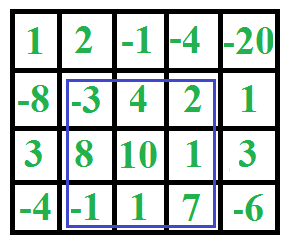Related Articles

# C# Program for Maximum sum rectangle in a 2D matrix | DP-27

• Last Updated : 12 Dec, 2018

Given a 2D array, find the maximum sum subarray in it. For example, in the following 2D array, the maximum sum subarray is highlighted with blue rectangle and sum of this subarray is 29.This problem is mainly an extension of Largest Sum Contiguous Subarray for 1D array.

## C#

 `// C# Given a 2D array, find the``// maximum sum subarray in it``using` `System;`` ` `class` `GFG {`` ` `    ``/** ``* To find maxSum in 1d array ``* ``* return {maxSum, left, right} ``*/``    ``public` `static` `int``[] kadane(``int``[] a)``    ``{``        ``int``[] result = ``new` `int``[] { ``int``.MinValue, 0, -1 };``        ``int` `currentSum = 0;``        ``int` `localStart = 0;`` ` `        ``for` `(``int` `i = 0; i < a.Length; i++) {``            ``currentSum += a[i];``            ``if` `(currentSum < 0) {``                ``currentSum = 0;``                ``localStart = i + 1;``            ``}``            ``else` `if` `(currentSum > result) {``                ``result = currentSum;``                ``result = localStart;``                ``result = i;``            ``}``        ``}`` ` `        ``// all numbers in a are negative``        ``if` `(result == -1) {``            ``result = 0;``            ``for` `(``int` `i = 0; i < a.Length; i++) {``                ``if` `(a[i] > result) {``                    ``result = a[i];``                    ``result = i;``                    ``result = i;``                ``}``            ``}``        ``}``        ``return` `result;``    ``}`` ` `    ``/** ``* To find and print maxSum, `` ``(left, top), (right, bottom) ``*/``    ``public` `static` `void` `findMaxSubMatrix(``int``[, ] a)``    ``{``        ``int` `cols = a.GetLength(1);``        ``int` `rows = a.GetLength(0);``        ``int``[] currentResult;``        ``int` `maxSum = ``int``.MinValue;``        ``int` `left = 0;``        ``int` `top = 0;``        ``int` `right = 0;``        ``int` `bottom = 0;`` ` `        ``for` `(``int` `leftCol = 0;``             ``leftCol < cols; leftCol++) {``            ``int``[] tmp = ``new` `int``[rows];`` ` `            ``for` `(``int` `rightCol = leftCol;``                 ``rightCol < cols; rightCol++) {`` ` `                ``for` `(``int` `i = 0; i < rows; i++) {``                    ``tmp[i] += a[i, rightCol];``                ``}``                ``currentResult = kadane(tmp);``                ``if` `(currentResult > maxSum) {``                    ``maxSum = currentResult;``                    ``left = leftCol;``                    ``top = currentResult;``                    ``right = rightCol;``                    ``bottom = currentResult;``                ``}``            ``}``        ``}`` ` `        ``Console.Write(``"MaxSum: "` `+ maxSum + ", ``        ``range: [(``" + left + "``, ``" + top + "``)(``" + right + "``, ``" + bottom + "``)]");``    ``}`` ` `    ``// Driver Code``    ``public` `static` `void` `Main()``    ``{``        ``int``[, ] arr = { { 1, 2, -1, -4, -20 },``                        ``{ -8, -3, 4, 2, 1 },``                        ``{ 3, 8, 10, 1, 3 },``                        ``{ -4, -1, 1, 7, -6 } };``        ``findMaxSubMatrix(arr);``    ``}``}`` ` `// This code is contributed``// by PrinciRaj1992`
Output:
```MaxSum: 29, range: [(1, 1)(3, 3)]
```

Please refer complete article on Maximum sum rectangle in a 2D matrix | DP-27 for more details!

My Personal Notes arrow_drop_up